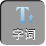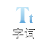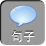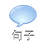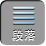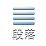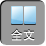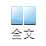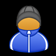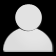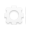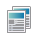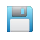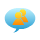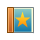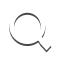-AA+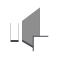1、多项式的乘法法则：多项式与多项式相乘，先用一个多项式的每一项乘另一个多项式的每一项，再把所得的积相加.

2、运用多项式乘法法则时的注意事项：

1）多项式的乘法法则，是两次运用单项式与多项式相乘的法则得到的.

( a+ b)( m+ n)=( a+ bm+( a+ bn

( a+ b)( m+ n)= am+ bm+ an+ bn.

2）多项式与多项式相乘，仍得多项式，在合并同类项之前，积的项数应等于两个多项式的项数之积，如( a+ b)( x+ y+ z)的项数在没合并前，应是 2× 3= 6项.

3）注意确定积中每一项的符号，多项式中每一项都包含它前面的符号.

4）多项式乘多项式的积中，有同类项要合并同类项.

5）在进行计算含有一个相同字母的两个一次二项式相乘时，可借助下列公式进行快速计算：( x+ a)( x+ b)= x 2+( a+ b) x+ ab.

3、典例分析：

( 2)( x-y)( x 2+ xy+ y 2)

= 2 ax+ 3 bx -4 ay -6 by.

( 2)原式= x· x 2+ x· xy+ x· y 2+( - yx 2+( - yxy+( - yy 2

= x 3+ x 2 y+ xy 2 - x 2 y-xy 2 - y 3

= x 3 - y 3.

2）乘积中有同类项，要合并同类项.

( a+ b) 2=( a+ b)( a+ b)= a 2+ 2 ab+ b 2

( a-b) 2=( a-b)( a-b)= a 2 -2 ab+ b 2

( 1)( 3 a+ 5 b) 2;

( 2)( - x+ 3 y) 2 ;

( 3)( - m -2 n) 2.

=( 3 a) 2+ 2.3 a. 5 b+( 5 b) 2

= 9 a 2+ 30 ab+ 25 b 2.

( 2)( - x+ 3 y) 2

=( 3 y-x) 2=( 3 y) 2 -2· 3 y· x+ x 2

= 9 y 2 -6 xy+ x 2.

( 3)( - m -2 n) 2

=[ -( m+ 2 n)] 2

=( m+ 2 n) 2

= m 2+ 4 mn+ 4 n 2.

2）完全平方公式( a± b) 2= a 2± 2 ab+ b 2，展开式可记成“首 (a)平方、尾 (b)平方，首 (a)(b)乘积的 2倍加减在中央”.

( 2)( a -2 b+ 3 c)( - a -2 b -3 c).

= 9 y 6 -4 x 4.

( 2)原式=[ -2 b+( a+ 3 c)][ -2 b -( a+ 3 c)]

=( -2 b) 2 -( a+ 3 c) 2

= 4 b 2 -( a 2+ 6 ac+ 9 c 2)

= 4 b 2 - a 2 -6 ac -9 c 2.

( 1) -3 x 2+ 6 x 2 y -3 xy 2;

( 2) x 4 -9 x 2.

= -3 x( x 2 -2 xy+ y 2)

= -3 x( x-y) 2.

( 2) x 4 -9 x 2

= x 2( x 2 -9)

= x 2( x+ 3)( x -3).

( 3)( m 2 -1) 2+ 6( m 2 -1)+ 9

=( m 2 -1) 2 -6( m 2 -1)+ 9

=( m 2 -1-3) 2

=[( m+ 2)( m -2)] 2

=( m+ 2) 2( m -2) 2.

2）多项式第一项为负时，要先提出“ -”号，使括号内的第一项为正.

3）因式分解必须彻底，即分解到不能再进行分解为止.